# AP Physics 1 Multiple-Choice Practice Test 34

### Test Information10 questions18 minutes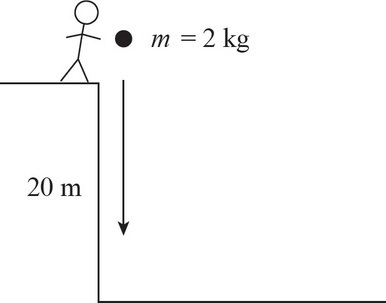1. A 2 kg rock is dropped off a cliff with a height of 20 m. What is the speed of the rock at the bottom of the hill?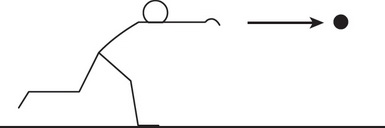2. How much work is done by a pitcher in throwing a 0.2 kg ball at a speed of 30 m/s ?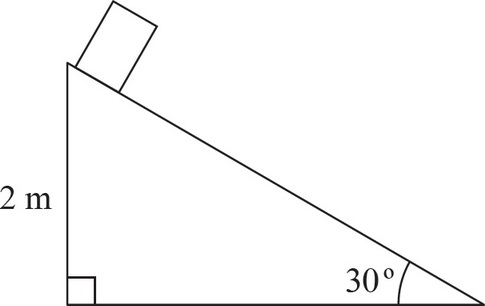3. A 10 kg block is placed at the top of an inclined plane with an angle of incline of 30° and μk = 0.1. If the height of the inclined plane is 2 m, what is the kinetic energy of the block when it is halfway down the incline?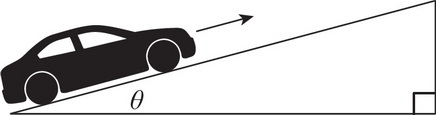4. A car's engine must exert a force of 2,000 N to maintain a speed of 30 m/s up an incline. What is the power provided by the engine during this motion?

5. Question below refers to the following scenario: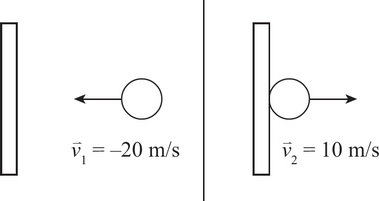A 2 kg ball with a velocity of -20 m/s collides with the wall and bounces back with a velocity of 10 m/s.

What is the impulse during the collision?

6. Question below refers to the following scenario:A 2 kg ball with a velocity of -20 m/s collides with the wall and bounces back with a velocity of 10 m/s.

If the ball is in contact with the wall for 0.002 s, determine the average force experienced by the ball.

7. Question below refers to the following scenario:A 2 kg ball with a velocity of -20 m/s collides with the wall and bounces back with a velocity of 10 m/s.

How much work did the wall do on the ball?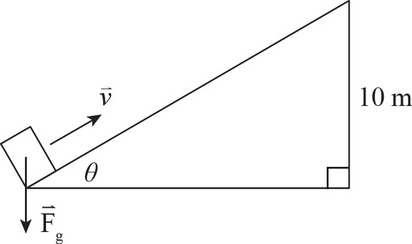8. A student pushes a 6 kg box up an inclined plane with a height of 10 m. How much work does gravity do on the box during this process?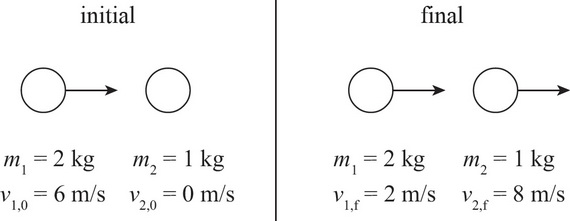9. A 2 kg ball traveling to the right at 6 m/s collides head on with a 1 kg ball at rest. After impact, the 2 kg ball is traveling to the right at 2 m/s and the 1 kg ball is traveling to the right at 8 m/s. What type of collision occurred?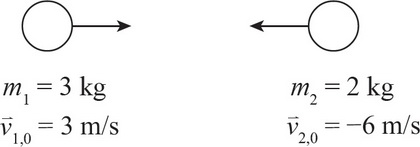10. A 3 kg mass with an initial velocity of +3 m/s has a perfectly inelastic collision with a 2 kg mass with an initial velocity of -6 m/s. What is the final velocity after impact?